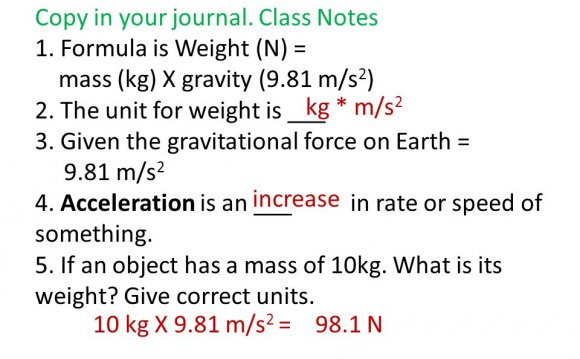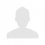# Formula for gravitational pull

October 26, 2023The water above receives energy as it falls down the short waterfall. This energy was stored as potential energy in the gravitational field of the Earth and came out of storage as the water dropped. This energy which came out of the gravitational field ended up being expressed as the kinetic energy of the water. That is, the water gains kinetic energy as it drops. An ounce of water is going faster when it hits the bottom of the waterfall than it was when it went over the top of the waterfall.

First Definition

Energy that is stored in the gravitational field is called gravitational potential energy, or potential energy due to gravity.

Formula For Work

Let's see how energy gets stored in the gravitational field.

First of all, remember that work equals force times distance as stated in this formula:

The above formula for work shows a dot product for the multiplication of the force vector times the displacement vector. If the force and displacement vectors are in the same direction, then this boils down to a simple multiplication of the size of the force times the size of the displacement. This will be the case for our discussion here.

Force Doing The Lifting

Now, let's consider what happens when an object is being lifted. We will consider lifting an object at constant velocity. That is, during the lift the object will not be accelerating. For the moment we will not discuss the very start of the lift, when the object must begin to move upward from a stationary position. Nor will we, for the moment at least, discuss the very end of the lift, when the object must slow to a stop after the upward motion is finished. Here, we are talking about the motion during the lift, and we will consider this motion to be directly upward and at constant speed.

If the object is being lifted at constant velocity, then it is not accelerating, and the net force on it is zero. We know that from Newton's first law of motion.

We must pull up on an object when we lift it. So, the agent doing the lifting must provide an upward force on the object. This would be the yellow applied force in the animation at left.

Gravity, of course, will be pulling down. This pull down of gravity is called the weight of the object. It is the blue downward force in the animation at left.

If during the lift the net force on the object is zero, then the upward pull must be canceled by the downward pull. That is, the upward lifting force must be equal in size to the downward pull of gravity.

In summary, when you are lifting something at constant velocity, the upward pull that you provide is equal to the weight of the object.

When lifting something at a constant velocity.

The force that you lift with (applied force)

Equals

The weight of the object (gravitational force)

Distance For The Lift

Over what distance does this force act? Well, that would be the distance over which you lift the object. That distance we will call the height of the lift.

Source: zonalandeducation.com

##### INTERESTING FACTS##### What is the formula for gravitational pull on the moon?

83.3% (or 5/6) of the gravity of Earth. The moon is about 1/4 the size of Earth. !